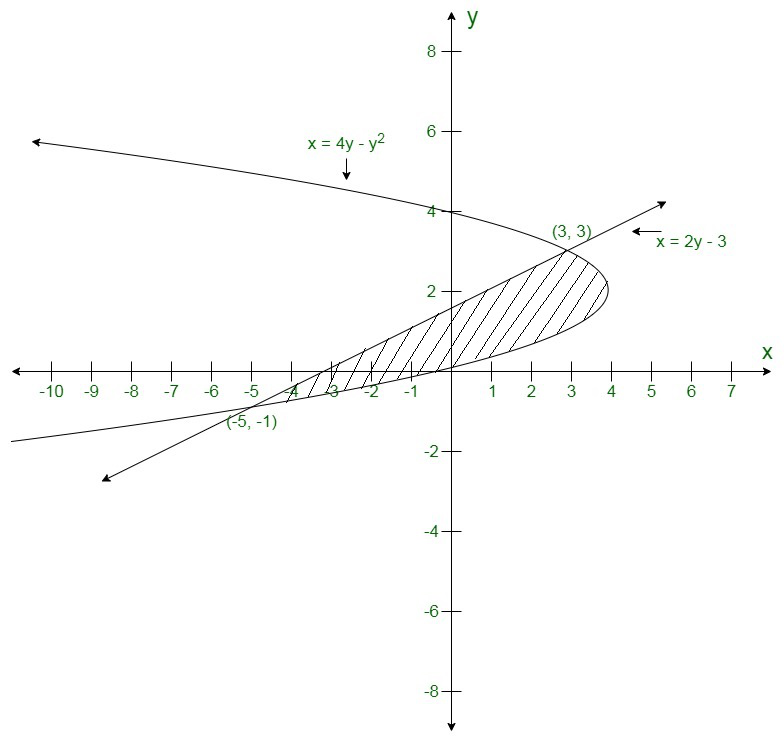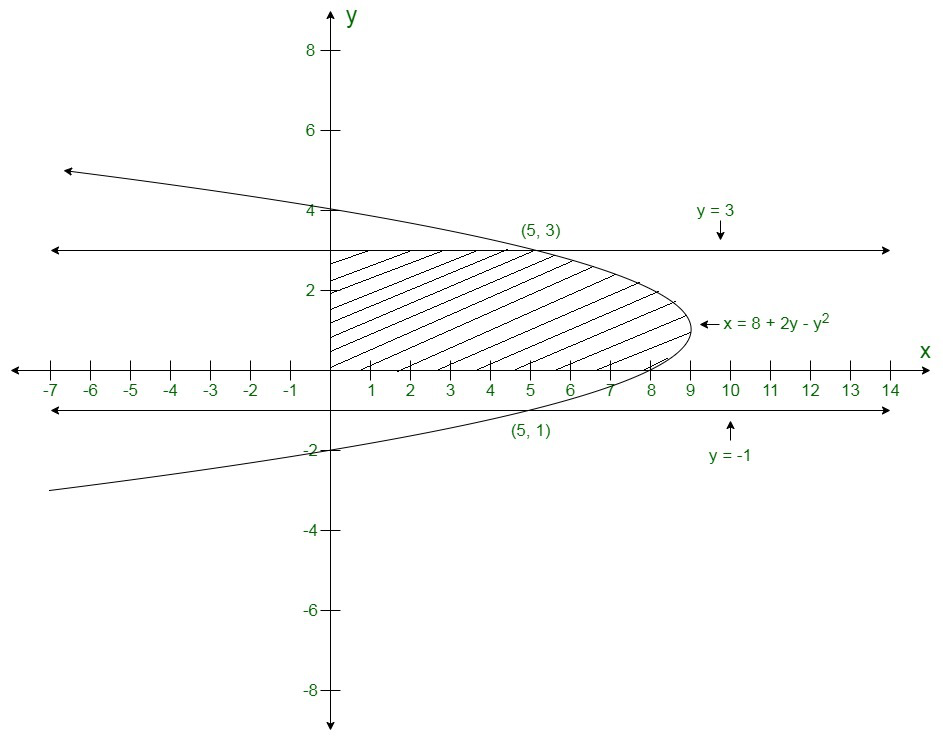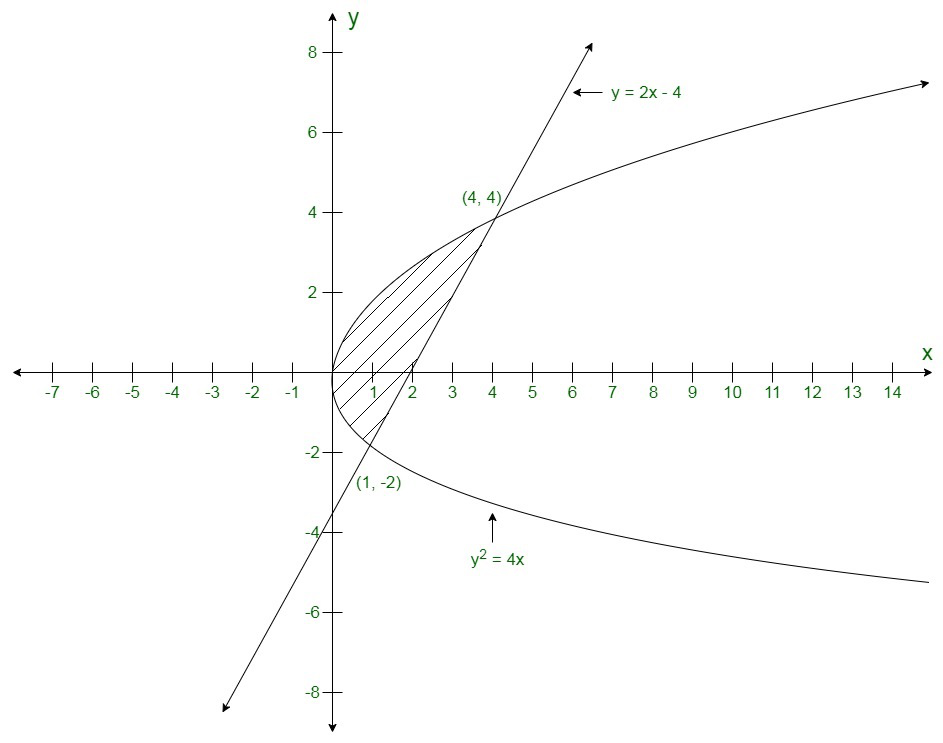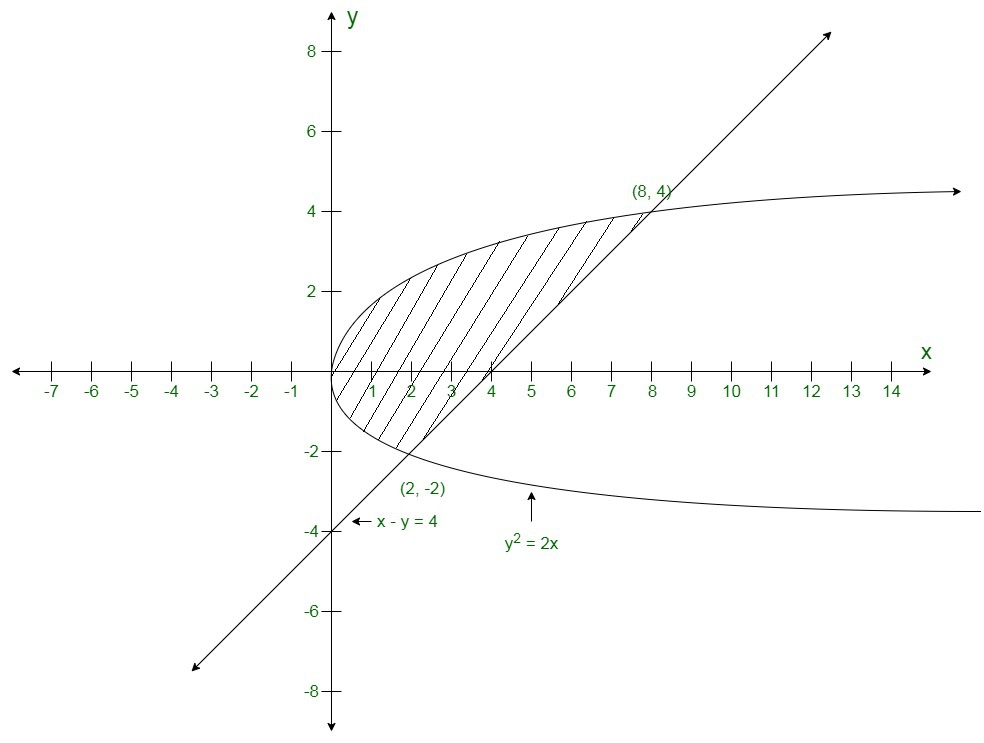Open in App
Not now

# Class 12 RD Sharma Solutions- Chapter 21 Areas of Bounded Regions – Exercise 21.4

• Last Updated : 19 Jan, 2021

### Question 1. Find the area of the region between the parabola x = 4y − y2 and the line x = 2y − 3.

Solution:Area of the bounded region### Question 2. Find the area bounded by the parabola x = 8 + 2y − y2; the y-axis and the lines y = −1 and y = 3.

Solution:Area of the bounded region### (ii) By using vertical strips

Solution:Area of the bounded region### Question 4. Find the area of the region bounded the parabola y2 = 2x and straight line x − y = 4.

Solution:Area of the bounded regionMy Personal Notes arrow_drop_up